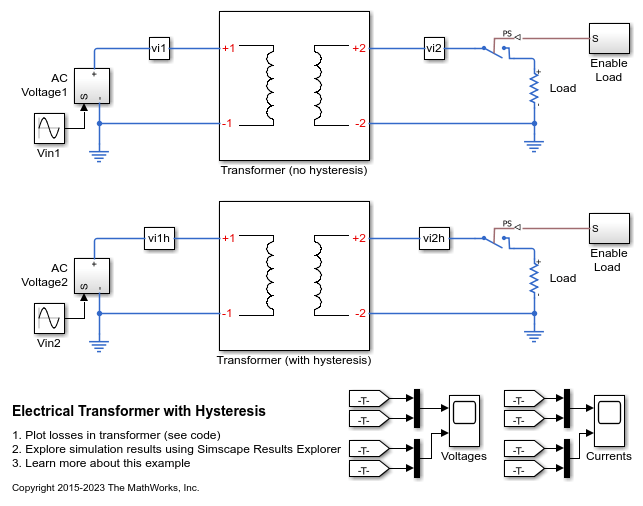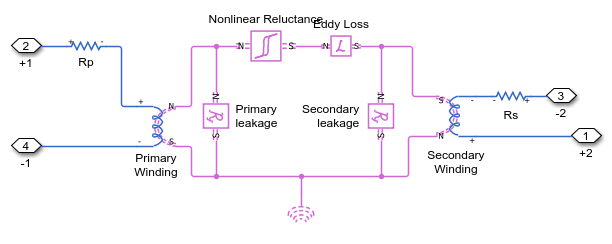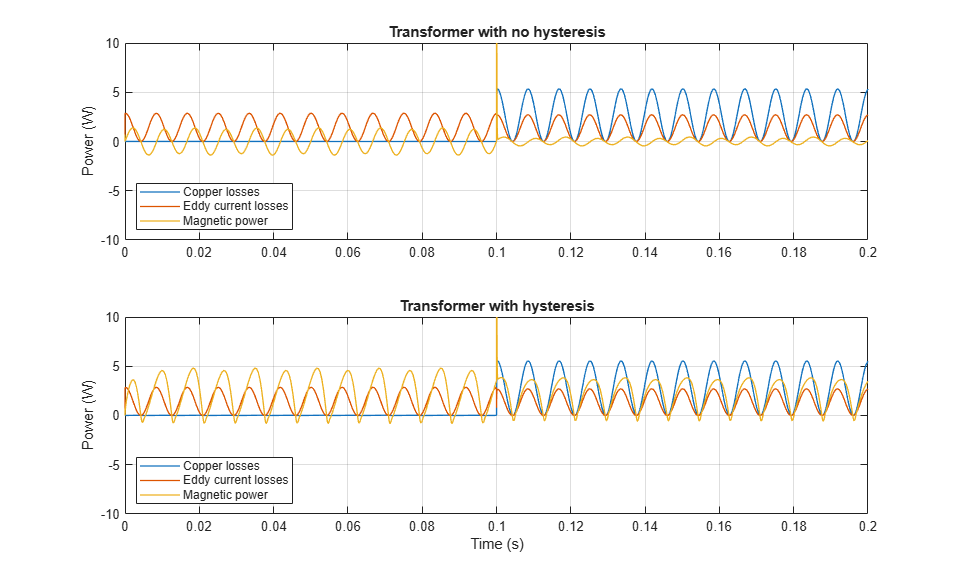# Electrical Transformer with Hysteresis

This example shows how to model a custom transformer that exhibits hysteresis by using the Non Linear Reluctance block in a magnetic circuit. The transformer is rated for a 50W load and steps down from 120V to 12V rms. The magnetizing resistance Rm is modeled in the magnetic domain using an Eddy Loss block.

The transformer initially operates under no-load conditions. At time t=0.05s, the rated load is turned on. For comparison purposes, the transformer is modeled with and without hysteresis by two separate subsystems. The primary and secondary inductance values L1 and L2 plus associated winding numbers N1 and N2 are used to determine the two leakage reluctances.

The magnetic hysteresis losses are determined by calculating the total electrical power flowing into the magnetic circuit from both windings. The difference between power in and power out over a complete AC cycle reflects the hysteresis and eddy losses. In order to obtain just the hysteresis loss, the eddy loss is subtracted from the total. The loss is calculated separately for the no-load and rated load conditions.

### Model### Transformer Subsystem### Simulation Results from Simscape Logging

```No-load hysteresis loss = 2.3111 watts Rated-load hysteresis loss = 2.2045 watts ```